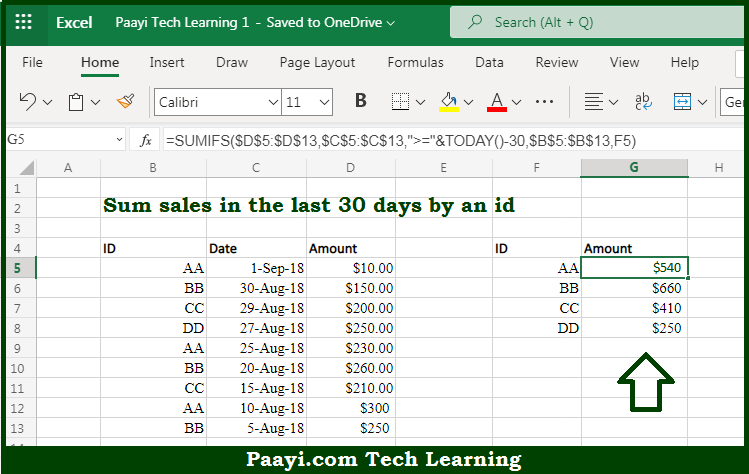# Learn How to SUM Sales in Last 30 Days By ID in Microsoft Excel

Written by | 0 Comments | 513 Views

In this article, you will learn how to SUM various things in Microsoft Excel using a single/combination(s) of functions. You will also know how to SUM Sales in the Last 30 Days By ID and see the generic formula.

SUM Sales in Last 30 Days By ID in Microsoft Excel

The main purpose of this formula is to sum sales in the last 30 days as per the id like - name, initials, region, etc. Here we will learn how to sum sales in the last 30 days by the given ID in the workbook in Microsoft Excel. That implies, with the help of a formula based on the SUMIFS and TODAY function you can able sum sales in the last 30 days according to ID like-  name, initials, region, etc. So, with the help of this formula, you can able to sum sales in the last 30 days by the given ID in the workbook in Microsoft Excel.

General Formula to SUM Sales in Last 30 Days By ID

=SUMIFS(amounts,dates,">="&TODAY()-30,ids,id)

The Explanation for the SUM Sales in Last 30 Days By IDSo we know that with the help of the given formula above you can able to sum sales in the last 30 days as per the id like - name, initials, region, etc. Here we will learn how to sum sales in last 30 days by the given ID in the workbook in Microsoft Excel. As we know that the SUMIFS function can is used to manage multiple criteria while calculating a sum. In the example provided here, the SUMIFS function is configured with a sum range for all amounts. It should be noted that the first criteria specify a criteria range that contains all dates, with a criterion greater than or equal to the current date minus 30. Similarly, the second criteria specify a criteria range of all IDs. That is with a criterion of a single ID pulled from the given column. So, with the help of this formula, you can able to sum sales in the last 30 days by the given ID in the workbook in Microsoft Excel.